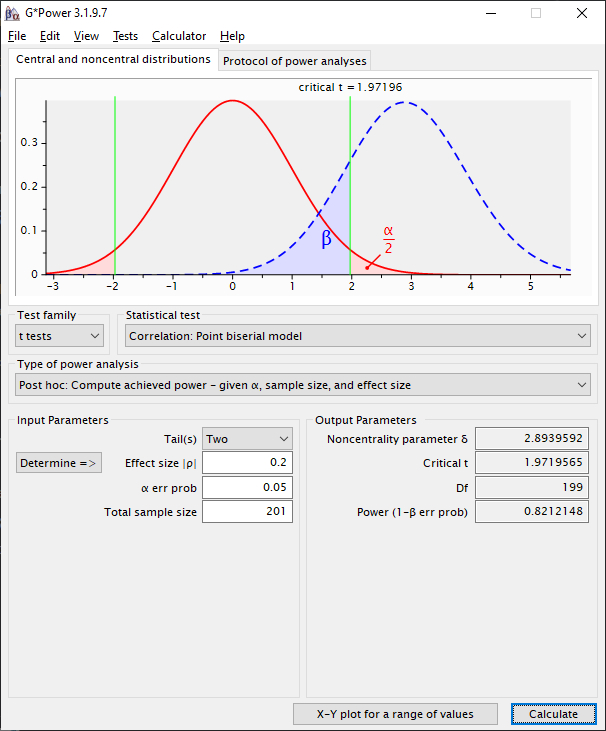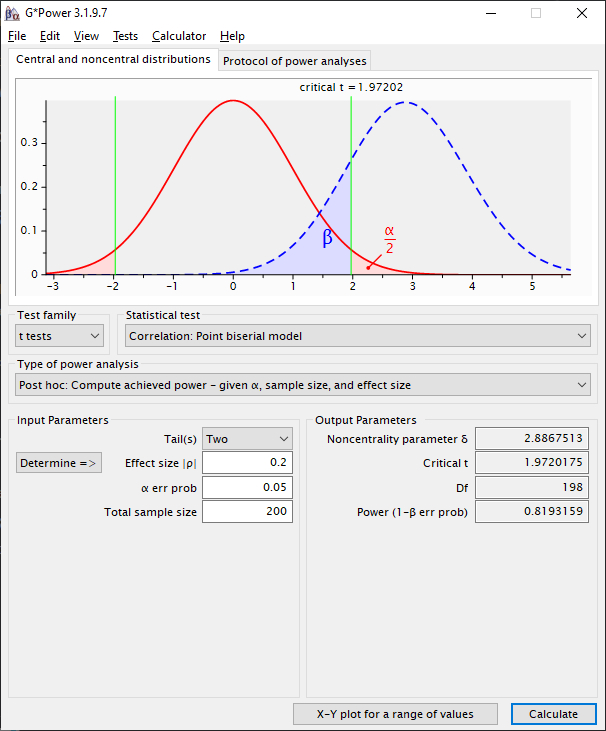# Frequently Asked Questions and Limitations

## Frequently Asked Questions

### How to Calculate Power

Power can be calculated using the power.rmcorr function. This function modifies pwr.r.test from the pwr package to use the rmcorr degrees of freedom. It is not presently included in the rmcorr package.

Notation: N is the sample size, k is the (average) number of repeated measures for each individual, and rrm is the rmcorr effect size.

#### power.rmcorr Example

N = 100, k = 3, and rrm = 0.20. This design has 82% power.

install.packages("pwr")
require(pwr)

power.rmcorr <- function(k, N, effectsizer, sig)
{pwr.r.test(n = ((N)*(k-1))+1, r = effectsizer, sig.level = sig)}

power.rmcorr(k = 3, N = 100, effectsizer = 0.20, sig = 0.05)
#>
#>      approximate correlation power calculation (arctangh transformation)
#>
#>               n = 201
#>               r = 0.2
#>       sig.level = 0.05
#>           power = 0.8156984
#>     alternative = two.sided

For G*Power or other software, power can be calculated by substituting the rmcorr degrees of freedom for a Pearson correlation. Instead of sample size, use the degrees of freedom for rmcorr plus two (effective sample size). This is because a Pearson correlation has N - 2 degrees of freedom.

• Rmcorr exact degrees of freedom = N x (k - 1) - 1
• Approximate degrees of freedom = (N -1) x (k - 1)

#### Exact Degrees of Freedom Example

Values: N = 100, k = 3 , and rrm = 0.20 (same as above)
rmcorr df = 100 x (3 - 1) - 1 = 200 - 1 = 199
Add two (kludge): 199 + 2 = 201
Enter N = 201 as the effective sample size in G*Power
G*Power will calculate the degrees of freedom as N - 2 = 201 - 2 = 199. Thus, using the correct degrees of freedom for rmcorr.

Note power is just slighty different than the same calculation in R, which uses an arctan approximation.G*Power using the exact degrees of freedom

#### Approximate Degrees of Freedom Example

Values: N = 100, k = 3, and rrm = 0.20 (again, same as above)
approx rmcorr df = (100 - 1) x (3 - 1) = 99 x 2 = 198
Note the small difference between the approximate vs. exact calculation: one degree of freedom
Add two (same kludge) for G*Power, entering a sample size of N = 200

Note power is slighty different than the exact formula above.### How to Extract the Slope and its Confidence Interval

my.rmc <- rmcorr(participant = Subject, measure1 = PaCO2, measure2 = pH,
dataset = bland1995)
#> Warning in rmcorr(participant = Subject, measure1 = PaCO2, measure2 = pH, :
#> 'Subject' coerced into a factor

# Structure of rmcorr object
#str(my.rmc)

# Extract rmcorr model coefficients
coef.rmc  <- my.rmc$model$coefficients
coef.rmc
#>  (Intercept) Participant1 Participant2 Participant3 Participant4 Participant5
#>   7.65590848  -0.72605418  -0.02144291   0.22395850   0.24550355   0.13432752
#> Participant6 Participant7     Measure1
#>   0.20037424  -0.03394863  -0.10832305

slope.rmc <- coef.rmc[length(coef.rmc)] #Last value in coefficients is the slope
slope.rmc
#>  Measure1
#> -0.108323

# Confidence intervals around all estimates
coef.CIs <- stats::confint(my.rmc\$model)
coefs.all <- cbind(coef.rmc, coef.CIs)
coefs.all
#>                 coef.rmc       2.5 %      97.5 %
#> (Intercept)   7.65590848  7.34994594  7.96187102
#> Participant1 -0.72605418 -0.83211999 -0.61998837
#> Participant2 -0.02144291 -0.11116705  0.06828123
#> Participant3  0.22395850  0.16049969  0.28741732
#> Participant4  0.24550355  0.16448903  0.32651806
#> Participant5  0.13432752  0.05868538  0.20996967
#> Participant6  0.20037424  0.12673292  0.27401556
#> Participant7 -0.03394863 -0.17148111  0.10358385
#> Measure1     -0.10832305 -0.16883787 -0.04780822

### isa Error

We have had reports of this error when running rmcorr:

Error in isa(Participant, "character") : could not find function "isa" 

Stack Question with error

Updating R to version 4.1.0 or later resolves this error.

### Transformations

Transformations can be used to make the data (errors) more normal. We highly recommend graphing both the raw and transformed data. It may be appropriate to only transform one measure or transform both measures. “Consider transforming every variable in sight” (Gelman & Hill, 2017: Data Analysis Using Regression and Multilevel/Hierarchical Models, ISBN: 9780521686891, p. 548): Google Books

## Limitations

### Change Over Time

In general, rmcorr is a time-independent model– it does not model change over time. A partial exception is if time is a measure, such as age in the raz2005 dataset.

### Non-Linearity

Rmcorr fits a linear model. If the data are non-linear, we recommend trying to transform it or using multilevel modeling.

### Varying Slopes with Influential Observations and/or Unbalanced Data

If slopes meaningfully vary by individual, we recommend using multilevel modeling instead of rmcorr. Random effect slopes are even more problematic for rmcorr with influential observations and/or highly unbalanced data. This is nicely illustrated in simulations by Dr. Marta Karas:
When rmcorr may not be ideal

## Other Implementations of rmcorr

We know of three other implementations of rmcorr.